﻿ Graph Spectrum - 演算法筆記Aᵀ可以翻轉所有邊的方向。

Vertex Labeling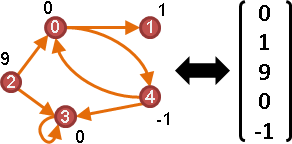Edge LabelingNeighbor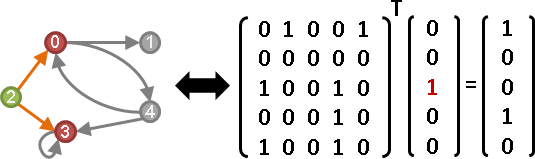Aᵀx。x的元素們，起點是1，其餘點是0。

Degree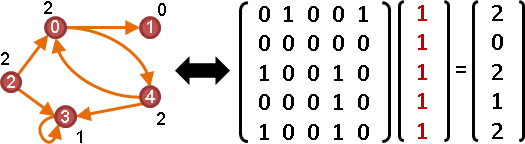Aᵀx是入邊數量，Ax是出邊數量。x的元素們，通通是1。

WalkAᴺ。N是步數。

Strassen's Algorithm需要用到元素加法反運算，然而布林OR沒有反運算。改弦易轍，以實數代替布林，零當作false，非零當作true。

Walk詳細解釋```r₂(i, k) = ( r₁(i, 0) AND r₁(0, k) ) OR
( r₁(i, 1) AND r₁(1, k) ) OR
( r₁(i, 2) AND r₁(2, k) ) OR
:
( r₁(i, V-1) AND r₁(V-1, k) )

r₂(i, k)：i點走到k點，是否剛好2條道路。
```

i點到j點，j點到k點，窮舉j點──宛如矩陣乘法求元素(i,k)。一次矩陣乘法就將所有(i,k)配對通通算好。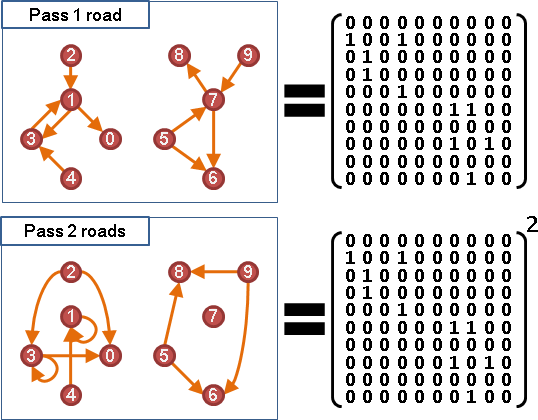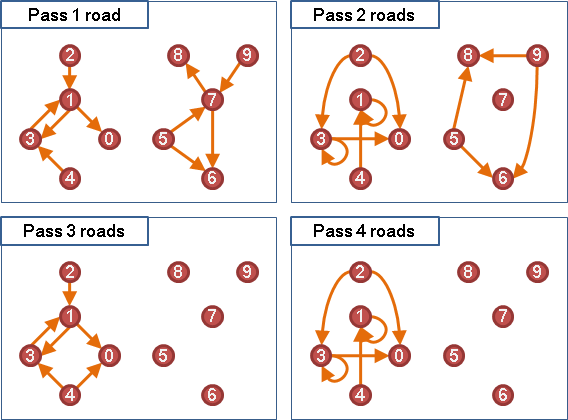UVa 10681

Transitive ClosureA* = I ⋁ A ⋁ A² ⋁ ... ⋁ A

A* = I ⋁ A ⋁ A² ⋁ ... ⋁ AV-1

（一）以實數代替布林。

A* = I + A + A² + ... + AV-1

（二）級數化作分式。

A* = I + A + A² + ... = (I - A)⁻¹

A* = I + (αA) + (αA)² + ... = (I - αA)⁻¹

UVa 12695

Strongly Connected Component(A* ⋀ A*ᵀ)x。往返，鄰居，一次找一個。

PathD = ∞I + W + W² + ... + WV-1

```http://theory.stanford.edu/~virgi/cs367/
http://www.lab2.kuis.kyoto-u.ac.jp/keisan-genkai/reports/2006/nhc/Uri_Zwick.pdf
```

Circuit```A¹對角線：一步回到原處。
A²對角線：兩步回到原處。
A³對角線：三步回到原處。
```
```A¹對角線總和：自環數量。
A²對角線總和：邊數的兩倍。（A預先刪除自環）
A³對角線總和：三角形數量的六倍。（A預先刪除自環）
```
```tr(A) = L                      loop number L
tr(A²) = 2E   when tr(A) = 0   edge number E
tr(A³) = 6T   when tr(A) = 0   triangle number T
```

Directed Acyclic GraphComponentrank(U) = V - C。無向圖的上三角矩陣（不含對角線），矩陣維度＝點數－連通分量數量。

MatchingTutte Matrix：權重換成變數。下三角矩陣變號。刪除對角線。

```Tᵢⱼ = { xᵢⱼ    if i < j and edge (i,j) exists
{ -xⱼᵢ   if i > j and edge (i,j) exists
{ 0      otherwise
```

rank(T)：最大匹配的邊數的兩倍。

det(T) ≠ 0：完美匹配是否存在。

Component

```http://web.cs.elte.hu/~lovasz/eigenvals-x.pdf
https://ilyaraz.org/static/class/scribes/scribe14.pdf
https://ilyaraz.org/static/class/scribes/scribe15.pdf
```

Random Walk

Laplacian Matrix

Laplace Operator「拉普拉斯運算子」。處處求得梯度的散度。

Laplacian Matrix「梯散矩陣」。拉普拉斯運算子，寫成矩陣L。

L模仿「Laplace Operator」，Lx模仿「中央與四周的差距總和（四周減中央）」。理應是L = A - D，卻誤植成L = D - A。圖論專家不知道是哪根筋不對勁。大家只好將錯就錯。L D A代表Laplacian Matrix、Degree Matrix、Adjacency Matrix。

Pairwise Product / Pairwise Squared DistanceAx是鄰點總和。

```一點：[x₁]             （無向圖、完全圖、有自環）

```

xᵀAx是兩兩相乘、通通加總。

```一點：x²

```

Lx是鄰邊總和：自己與鄰點的差距，填在邊上。

```一點：[x₁-x₁]

```

xᵀLx是兩兩相減平方、通通加總。

```一點：0

```

```Ax   = [ ∑ⱼ aᵢⱼ xⱼ ]                      鄰點總和
xᵀAx = ∑ᵢ ∑ⱼ aᵢⱼ xᵢ xⱼ                    點對相乘總和
Lx   = [ ∑ⱼ aᵢⱼ (xᵢ - xⱼ) ]               鄰點與自身差異總和（無向圖）
xᵀLx = 1/2 ∑ᵢ ∑ⱼ aᵢⱼ (xᵢ - xⱼ)²           點對相減平方總和（無向圖）
(Dout-A)x        = [ ∑ⱼ aᵢⱼ (xᵢ - xⱼ) ]   鄰點與自身差異總和（有向圖）
xᵀ(Din+Dout-2A)x = ∑ᵢ ∑ⱼ aᵢⱼ (xᵢ - xⱼ)²   點對相減平方總和（有向圖）
```

Dirichlet Energy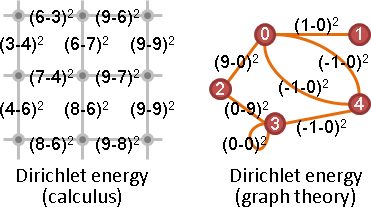「狄利克雷能量」。各處梯度長度平方和。等於xᵀLx。

```  sum ‖grad xᵢ‖²
= ½ sum sum Aᵢⱼ (xᵢ - xⱼ)²
= xᵀLx
```

【尚無正式名稱】「對應梯度差異平方和」。等於(x-y)ᵀL(x-y)。

```  sum ‖grad xᵢ - grad yᵢ‖²
= ½ sum sum Aᵢⱼ [(xᵢ - xⱼ) - (yᵢ - yⱼ)]²
= ½ sum sum Aᵢⱼ [(xᵢ - yᵢ) - (xⱼ - yⱼ)]²
= (x-y)ᵀL(x-y)
```

Cutmin xᵀLx。x的元素們，只能是0或1，不能全0全1。

Partition

《Minimal Dirichlet Energy Partitions for Graphs》

I haven't spent my skill points on inequality.

Random Walk《Random Walk st-Connectivity》

I haven't spent my skill points on inequality.

Isomorphism《Spectral Graph Isomorphism》

I haven't spent my skill points on inequality.

Laplacian Spectrum

Laplacian Spectrum

Laplacian Matrix的V個特徵值。

Spanning Tree

Commute-Time Distance

d(i,j) = L⁺ᵢᵢ + L⁺ⱼⱼ - 2L⁺ᵢⱼ。其中L⁺是虛擬反矩陣。

```https://math.stackexchange.com/questions/1679597/
https://math.stackexchange.com/questions/1321305/
```

Algebraic Connectivity

```http://web.stanford.edu/~boyd/papers/cvx_opt_graph_lapl_eigs.html
```

Incidence Matrix

Incidence Matrix（Differential Matrix）「關聯矩陣」、「微分矩陣」。點邊關係。不討論自環。

Laplacian MatrixL = MMᵀ。L代表無向圖的Laplacian Matrix，M代表有向圖的Incidence Matrix。

Componentrank(M) = V - C。矩陣維度＝點數－連通分量數量。Treedet(submatrix(V-1)×(V-1)(M)) = ±1。樹的Incidence Matrix，隨便砍掉一個橫條，行列式一定是+1或-1。Spanning TreeLaplacian Matrix的任意一個cofactor的行列式，等於生成樹數量。此性質稱作「Matrix Tree Theorem」。

cofactor就是隨便砍掉一個直條與一個橫條，剩下來的矩陣。根據砍掉的位置，乘上係數+1或-1 。請參考維基百科「Minor」。

L砍掉一個直條與一個橫條是L'。M砍掉一個橫條是M'。

L = MMᵀ。L' = M'M'ᵀ。det(L') = det(M'M'ᵀ)。

Cauchy–Binet公式，展開det(M'M'ᵀ)，得到許多(V-1)×(V-1)方陣的行列式，兩兩自乘、通通加總。